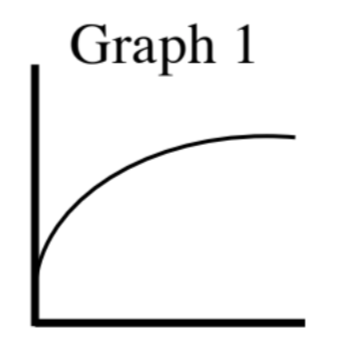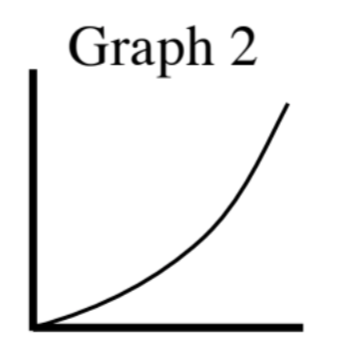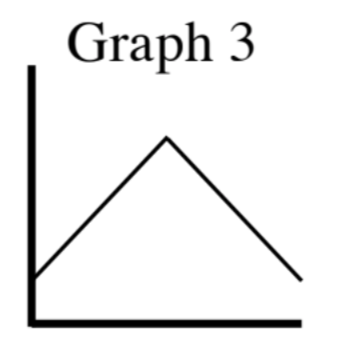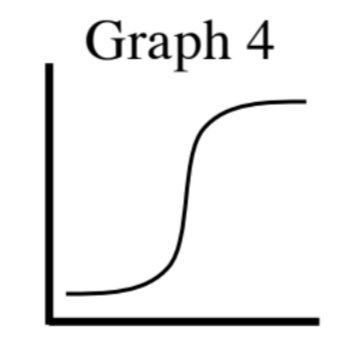### Home > INT2 > Chapter 5 > Lesson 5.1.2 > Problem5-23

5-23.

Match each table of data below with the most appropriate graph, and then briefly explain how you know the graph matches the data.

1. The temperature in an oven when cooking a roast.

Time (min)

Temperature ($º\text{F}$)

$0$

$70$

$5$

$200$

$15$

$300$

$30$

$325$

$60$

$325$

$120$

$325$

$180$

$325$

The temperature rises immediately but as time passes it levels-off.

Graph 1

1. The time needed for an athlete to run a certain distance.

Distance (meters)

Time (min:sec)

$100$

0:12

$200$

0:25

$400$

1:10

$800$

2:24

$1600$

5:20

$3000$

10:59

Graph 2